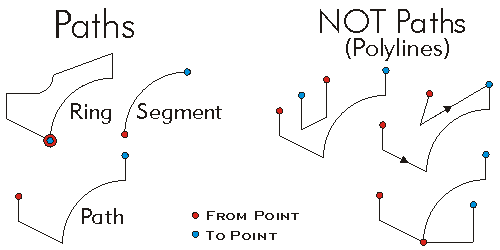ArcObjects Library Reference (Geometry)

# IPath Interface

Provides access to members that identify a path and define its behavior.

#### Product Availability

Available with ArcGIS Engine, ArcGIS Desktop, and ArcGIS Server.

#### Description

A Path is a connected, continuous sequence of Segments.  Except for the first and last Segments in the Path, each segment shares its FromPoint with the ToPoint of the previous Segment, and shares its ToPoint with the FromPoint of the following Segment.

#### Members

DescriptionDimension The topological dimension of this geometry.Envelope Creates a copy of this geometry's envelope and returns it.FromPoint The 'from' point of the curve.Generalize Generalizes this path using the Douglas-Poiker algorithm.GeometryType The type of this geometry.GeoNormalize Shifts longitudes, if need be, into a continuous range of 360 degrees.GeoNormalizeFromLongitude Normalizes longitudes into a continuous range containing the longitude. This method is obsolete.GetSubcurve Extracts a portion of this curve into a new curve.IsClosed Indicates if 'from' and 'to' points (of each part) are identical.IsEmpty Indicates whether this geometry contains any points.Length The length of the curve.Project Projects this geometry into a new spatial reference.QueryChordLengthTangents Returns tangent vectors (relative to corresponding endpoint) at both sides of a Bezier end point; and whether they have been set by user or by smoothing process.QueryEnvelope Copies this geometry's envelope properties into the specified envelope.QueryFromPoint Copies this curve's 'from' point to the input point.QueryNormal Constructs a line normal to a curve from a point at a specified distance along the curve.QueryPoint Copies to outPoint the properties of a point on the curve at a specified distance from the beginning of the curve.QueryPointAndDistance Finds the point on the curve closest to inPoint, then copies that point to outPoint; optionally calculates related items.QueryTangent Constructs a line tangent to a curve from a point at a specified distance along the curve.QueryToPoint Copies the curve's 'to' point into the input point.ReverseOrientation Reverses the parameterization of the curve ('from' point becomes 'to' point, first segment becomes last segment, etc).SetChordLengthTangents Sets tangent vectors (relative to corresponding endpoint) at both sides of a Bezier end point; if either is Nothing, they will be set by smoothing process.SetEmpty Removes all points from this geometry.Smooth Converts this path into a smooth approximation of itself that contains only Bezier curve segments.SmoothLocal Replaces up to four segments (two on each of the specified vertex index) with bezier curves.SnapToSpatialReference Moves points of this geometry so that they can be represented in the precision of the geometry's associated spatial reference system.SpatialReference The spatial reference associated with this geometry.ToPoint The 'to' point of the curve.

#### Inherited Interfaces

Interfaces Description
ICurve Provides access to properties and methods of all 1 dimensional curves (polylines, segments, boundaries of polygons, etc.).
IGeometry Provides access to members that describe properties and behavior of all geometric objects.

#### CoClasses that implement IPath

CoClasses and Classes Description
Path A sequence of connected segments.
Ring An area bounded by one, closed sequence of connected segments; optionally has measure, height and ID attributes at each vertex.

#### Remarks

The Segments composing the Path do not need to be homogenous in type.  A Ring is a Path that starts and ends on the same point and defines an area.  Paths are the part types in Polylines.Geometry service